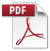# METERED PDU’S

### Power

INTELLIGENT POWER STRIPS

CANNON “MD” Metered PDU’s are rackmount industrial grade PDU for mission critical Data Centre’s.

• Our Metered PDU allows true RMS current monitoring via a simple digital RMS ammeter.
• Helps prevent circuit overloads typically during equipment installation and allows planning on actual real time power load.
• Available in both Horizontal and Vertical Versions

## Order Codes

 CANNON POWER DIRECTION SOCKET QTY SOCKET TYPE SOCKET QTY SOCKET TYPE 2 SOCKET QTY SOCKET TYPE 3 RATING MODEL TYPE CORD LENGTH INPUT PLUG FEED INPUT COLOUR CP V 02 3 = UK 45 02 1 = C13 02 2 = C19 1 = 13A 0 = BASIC 1 = 1m 1 = 60309 1=bottom 1=black H 04 4 = UK 135 04 04 2 = 16A 1 = MD 2 = 2m 2 = C20 2=top 2=blue 06 5 = UK 90 06 06 3 = 32A 2 = MT 3 = 3m 3 = UK 3=red 08 6 = CEE 7/4 08 08 4 = 10A 3 = MTS 4 = 4m 4 = Schuko 4=grey 10 7 = US NEMA 10 10 5 = 20A 4 = W 5 = 5m 5=UNTERMINATED 12 8 = CEE 7/5 12 12 6 = 30A 5 = WI 6 = C14 16 14 7 = 16A(3) 6 = WS 7=L6-20P 20 16 8 = 32A(3) 7 = WSI 8=LC6-30P 24 18 20 24 28 32 36

## Related Case Studies

There are no related case studies yet.

METERED PDU